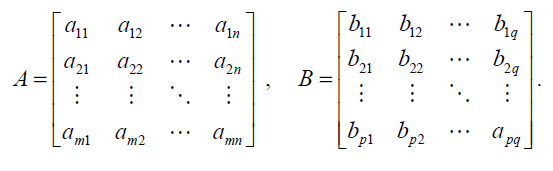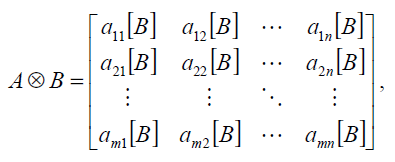## Problem S3: Quantum Operations

Quantum computing is currently a hot topic in research. If they can be built, quantum computers will have the ability to perform certain computing tasks much faster than any computer in existence today. Fortunately, you won't need a quantum computer to do this question.

A fundamental concept in quantum computing is the idea of as a quantum operation. A quantum operation can be essentially thought of as a matrix. Also, if you perform two quantum operations in parallel on separate quantum data, this can be thought of a larger quantum operation. Thinking of these operations in terms of matrices again, the resulting matrix from performing two matrices in parallel is called the tensor product of the two matrices (using the symbol ⊗). This is different from the normal product of matrices that you may have learned about.

In a tensor product, you are given two matrices (which are essentially two-dimensional arrays). We will call them A and B, and we will represent the individual elements of these two matrices this way:Notice that the size of matrix A is m×n (m rows and n columns), and the size of B is p×q.

The tensor product of these two matrices will be an mp×nq matrix (with mp rows and nq columns) that looks like:where aij[B] simply indicates that each element in B is being multiplied by aij.

Finally notice that the tensor product is not commutative, which means that changing the order of matrices may change the answer (A ⊗ B ≠ B ⊗ A).

For more than two matrices, we will define A ⊗ B ⊗ C = (A ⊗ B) ⊗ C , although it does not technically matter, since the tensor product is associative.

Your task is to compute and output the tensor product of two or more given matrices.

### Input

The first line of input contains the number of matrices, N, a positive integer. Then, there are N blocks of lines describing the matrices in order.

In each block, the first line will contain two positive integers, r and c, separated by a space, indicating the number of rows and columns, respectively. Then, the next r lines will denote the rows, in order. Each line will contain c integers, separated by spaces.

### Output

The output will be 6 integers in the following order:

• the maximum element in the tensor product
• the minimum element in the tensor product
• the maximum row sum (i.e., sum of entries in each row)
• the minimum row sum
• the maximum column sum
• the minimum column sum

You may assume that tensor product matrix will have no more than 1024 rows and no more than 1024 columns.

```2
2 2
1 1
1 -1
2 2
1 0
0 1```

```1
-1
2
0
2
0```

### Actual Tensor Product

```1 0 1 0
0 1 0 1
1 0 -1 0
0 1 0 -1```

```3
2 2
1 0
0 3
2 2
1 1
1 -1
2 2
1 0
0 1```

```3
-3
6
0
6
0```

### Actual Tensor Product

```1 0 1 0 0 0 0 0
0 1 0 1 0 0 0 0
1 0 -1 0 0 0 0 0
0 1 0 -1 0 0 0 0
0 0 0 0 3 0 3 0
0 0 0 0 0 3 0 3
0 0 0 0 3 0 -3 0
0 0 0 0 0 3 0 -3```

Point Value: 10
Time Limit: 2.00s
Memory Limit: 16M